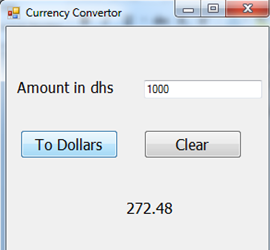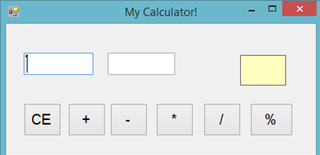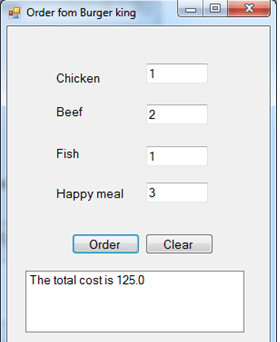# Exercise-2: Currency convertor and Calculator

### Practice Exercices

#### Question-1: Currency Convertor

1. Create a program and call it Currency_Convertor
2. Change the Name property of each control
3. Create a Clear Button
4. The user will type in the Amount in dirhams and clicks the “To Dollars” button
5. Then the program will convert form dirham to dollars where dollar = dirham / 3.67
7. Here is a guide:#### Question-2: Calculator

1. Create a program and call it Calculator
2. Change the Name property of each control
3. Add a code for each button to do the calculation and show the output in the result label:
• - Subtraction
• * Multiplication
• / Division
• % Reminder after dividing
4. Create a CE (Clear) Button
5. Add 4 comment to each Button
6. Here is a guide:#### Question-3: Burger King

1. Create a program and call it Burger_king as shown here:2. Change the Name property of each control
3. The user will type in:
• Number of Chicken Meals 20 dhs
• Number of Beef Meals 22 dhs
• Number of Fish Meals 25 dhs
• Number of Kids Meals 12 dhs
4. The program will calculate the Cost
5. The program will show the cost in a listbox